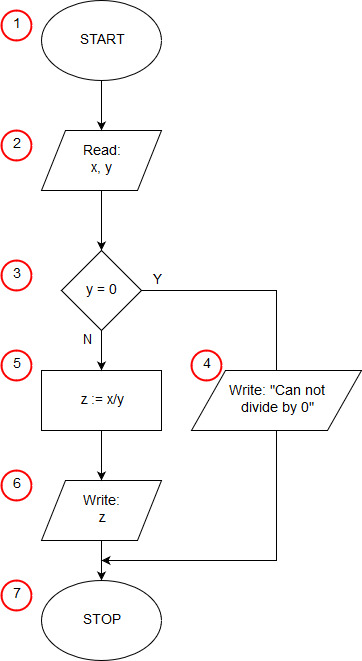# Algorithm of division in Java

<<algorithmimport java.util.Scanner; public class Divide { public static void main(String[] args) { float x,y; Scanner klaw=new Scanner(System.in); System.out.print("x="); x=klaw.nextFloat(); System.out.print("y="); y=klaw.nextFloat(); if(y==0) System.out.println("Can not divide by 0"); else { float z; z=x/y; System.out.print("x/y="); System.out.println(z); } } }

In the first line of the program, we inform you that we will use the Scanner class from the util java library. The Scanner class will be used to load input data in our program. In java, program execution starts with calling the public main() function contained in the class whose name matches the program name (the program must be saved in the Divide.java file and then compiled to the Divide.class file). definition class Divide starts in the 2nd program line. In our example, the main() function is the only function of the program. Line 3 contains the beginning of the main function definition, this is the beginning of the implementation of our algorithm (block START (1)). In the next line, we declare two variables x, y, which we will be using in a moment. In Java, variables can be declared anywhere before they are used, so we do not declare a variable here at once, which we may not need at all. In 5 lines, we declare and initialize the key variable as a Scanner object. We indicate that the source of text data will be the System.in stream, or keyboard.

Lines 6 through 9 perform an input/output (2) operation in which the input data is taken - a dividend one and a divisor.

The conditional statement from line 10 is the selection block (3) from our algorithm. The instruction checks whether y is equal to 0. When the condition is satisfied, the instruction stored in line 11 is executed (in the algorithm of the input input block (4)). When the condition is not met, the statement after the else keyword is executed, in our case it is a compound statement, enclosed in logical brackets {} between lines 13 and 16. First, on line 13, we declare a variable with, now we know that we will needed, then in line 14 we calculate the quotient x by y (operating block (5)), then in lines 15 and 16, print the result obtained (input-output block (6)).

The curly brace } from line 18 completes the main function and also the operation of the entire program (end block of the algorithm (7)), while the curly bracket in line 19 ends the definition of the Division class.